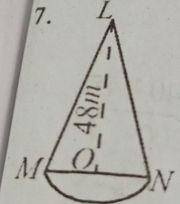### Mathematics Past Questions

4286

(a) Copy and complete the table of values for the relation y = 3 sin 2x.

 x o$$^o$$ 15$$^o$$ 30$$^o$$ 45$$^o$$ 60$$^o$$ 75$$^o$$ 90$$^o$$ 105$$^o$$ 120$$^o$$ 135$$^o$$ $$^o$$ y 0.0 1.5 -2.6

(b) Using a scale of 2 cm to 15° on the x-axis and 2cm to I unit on the y-axis, draw the graph of y = 3 sin 2x for 0° $$\geq$$ x $$\geq$$ 150°.

(c) Use the graph to find the truth set of;

(i) 3 sin 2x + 2 = 0;

(ii ) $$\frac{3}{2}$$ sin 2x = 0.25.

WAEC 2020
4287(a) The diagram shows a wooden structure in the form of a cone, mounted on a hemispherical base. The vertical height of the cone is 48 m and the base radius is 14. Calculate, correct to three significant figures, the surface area of the structure, [Take $$\pi = \frac{22}{7}$$]

(b) Five years ago, Musah was twice as old as Sesay. If the sum of their ages is 100, find Sesay's present age.

WAEC 2020
4288

(a) Ms. Maureen spent $$\frac{1}{4}$$ of her monthly income at a shopping mall, $$\frac{1}{3}$$ at an open market and $$\frac{2}{5}$$ of the remaining amount at a Mechanic workshop. If she had N222,000.00 left, find:

(i) her monthly income.

(ii) the amount spent at the open market.

(b) The third term of an Arithmetic Progression (A. P.) is 4m - 2n. If the ninth term of the progression is 2m - 8n. find the common difference in terms of m and n.

WAEC 2020
4289

(a)  Two cyclists X and Y leave town Q at the same time. Cyclist X travels at the rate of 5 km/h on a bearing of 049° and cyclist Y travels at the rate of 9 km/h on a bearing of 319°.

(a) Illustrate the information on a diagram.

(b) After travelling for two hours, calculate. correct to the nearest whole number, the:

(i) distance between cyclist X and Y;

(ii) bearing of cyclist X from Y.

(c) Find the average speed at which cyclist X will get to Y in 4 hours.

WAEC 2020
4290

The table shows the distribution of marks obtained by students in an examination.

 Marks (%) 0 - 9 10 - 19 20 -  29 30 - 39 40 - 49 50 - 59 60 - 69 70 - 79 80 - 89 90 - 99 Frequency 7 11 17 20 29 34 30 25 21 6

(a) Construct a cumulative frequency table for the distribution.

(b) Draw the cumulative frequency curve for the distribution.

(c) Using the curve, find correct to one decimal place, the:

(i) median mark;

(ii) lowest mark for the distinction if 5% of the students passed with distinction

WAEC 2020